# Topological Sort Algorithm

Learn about Topological Sort Algorithm.

Updated - 25 May 202223 mins readPublished : 5 Jan 2022
Published : 5 Jan 2022Challenge Inside! : Find out where you stand! Try quiz, solve problems & win rewards!

## Overview

Topological Sorting or Kahn's algorithm is an algorithm that orders a directed acylic graph in a way such that each node appears before all the nodes it points to in the returned order, i.e. if we have a --> b, a must appear before b in the topological order.

It's main usage is to detect cycles in directed graphs, since no topological order is possible for a graph that contains a cycle. Some of it's uses are: deadlock detection in OS, Course schedule problem etc.

## Scope

• This article tells about the working of the Topological Sorting.
• Need of Topological Sorting.
• Different operations of Topological Sorting.
• Implementation of Topological Sorting.
• Complexity of Topological Sorting.

# Takeaways

• Complexity of Topological Sorting
• Time complexity - $O(N + M)$ Where N is number of nodes and M is number of edges

## Introduction to Topological Sort

Today, before we talk about topological sort, let's talk about cake. Yes, cake. We're going to bake chocolate cake today.

So, what are the steps you follow for baking a cake?

1. You have certain ingredients, such as flour, baking powder, baking soda, yogurt, oil, etc. Before putting all these in the baking I'm sure you know that you must mix all these ingredients well.
2. But, before you can actually put the batter (mixed ingredients) into the pan, the pan must first be greased and floured properly.
3. Once you've poured the batter, you know that you need to now bake it. But then again, before you can bake the cake, you must preheat the oven.
4. To ice the cake, it must be cool. But to cool it down you must bake it first.
5. The cake has to be cooled before you can actually ice it.

Why this talk about baking cakes? Weren't we studying topological sort? Hold on, we're getting to it.

This complete procedure mentioned above can be represented as a graph. More specifically, a directed graph. Why? We mentioned that we must perform a particular action before going to the next action.

Basically, we have an order that must be followed, so it surely makes sense if the graph is directed, with each node's content being a particular action.

Here's how the graph might look like: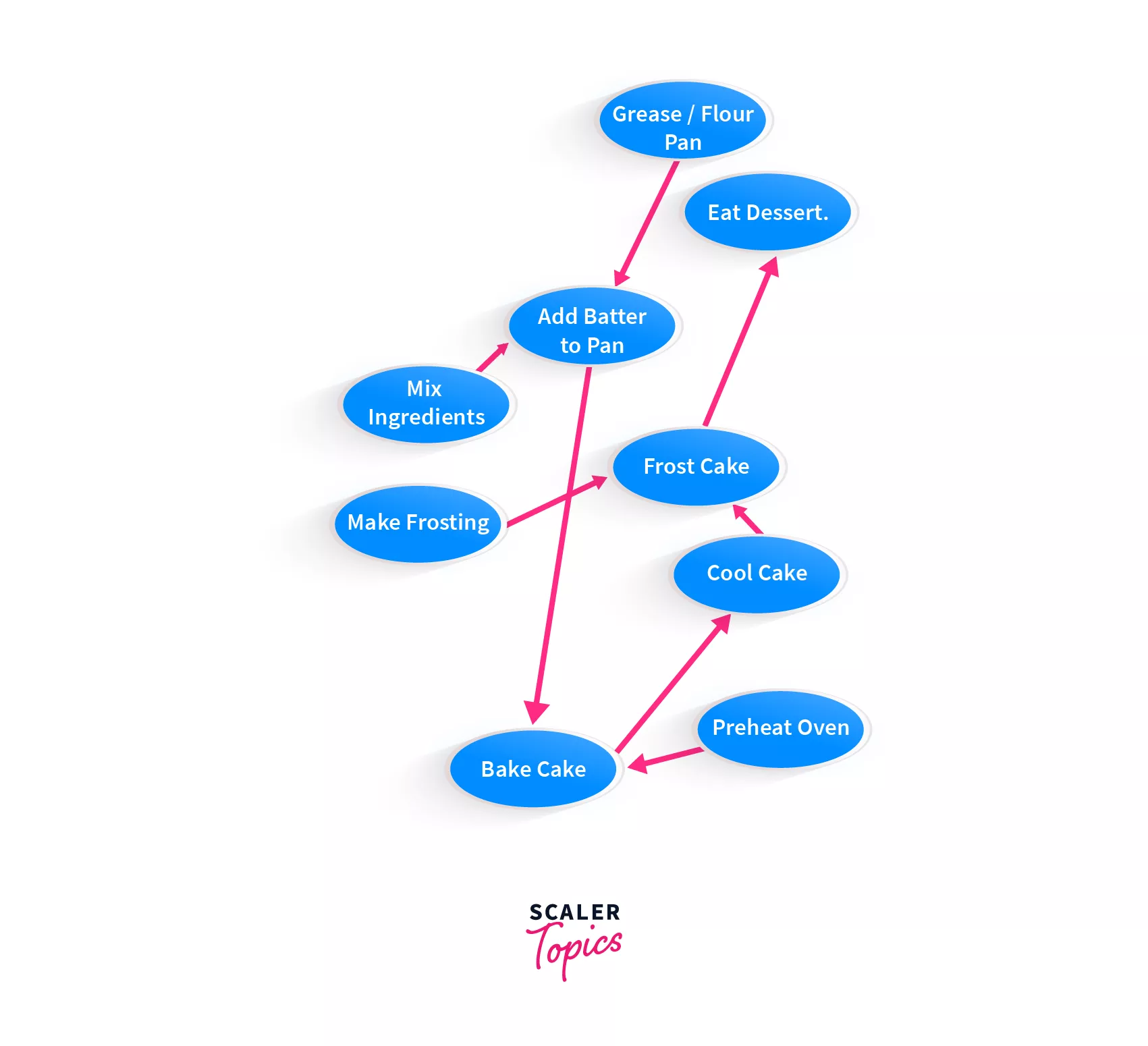As you can see in the above graph, you first need to mix the ingredients, and of course only after that you can add the batter to pan. Before baking the cake, you must grease the pan and so on.

What's the observation that we can make here? A graph can be sorted, by order of precedence of the nodes, and that is exactly what topological sort is!

We have set a foundation for studying topological sort, and we can now formally define it.

## What is Topological Sort?

Essentially, topological sort is an algorithm which sorts a directed graph by returning an array or a vector, or a list, that consists of nodes where each node appears before all the nodes it points to.

Here, we'll simply refer to it as an array, you can use a vector or a list too.

Say we had a graph,

a --> b --> c


then the topological sort algorithm would return - [a, b, c]. Why? Because, a points to b, which means that a must come before b in the sort. b points to c, which means that b must come before c in the sort.

Let's look at a relatively more complex example.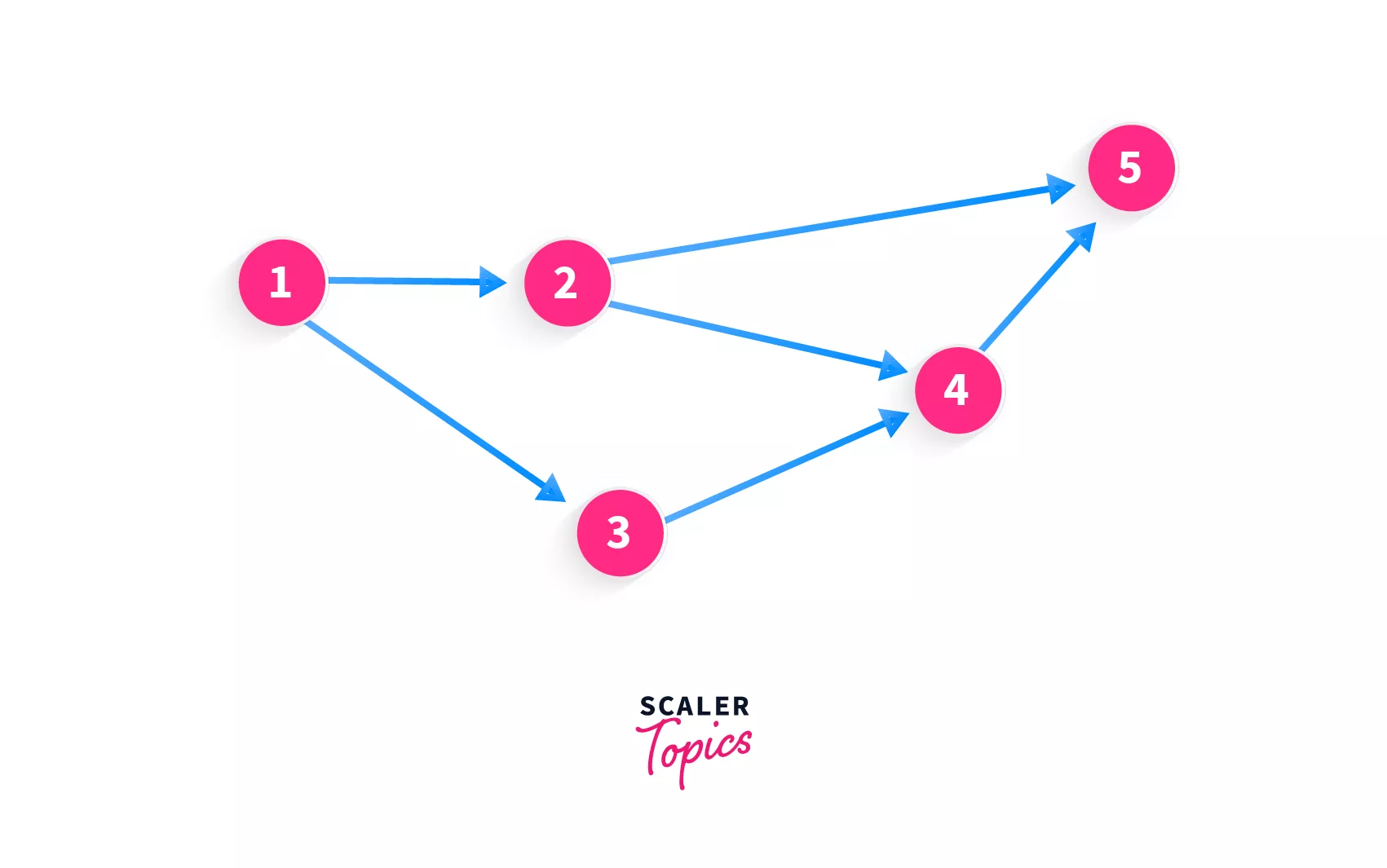What do you think the topological sort for this directed graph look like? Well, let's see. Initially, our return array / vector, or the sorted array / vector (array containing nodes of the graph in sorted order) is empty [].

Can we add 4 to the start of the sorted result? No! The algorithm states that if there are any nodes pointing to the current node, then they must be added first. So, if we want to add 4 to the sorted result, we must check out the node(s) that point to 4.

We can see that there are 2 nodes that can be added to the sorted result - 2, 3. Hold on, there is a node that points to them as well! So it all starts from the node with the value 1! Before we can add 2 or 3, we must add 1 because both the nodes (2, 3) have another node pointing to them, which means that neither of 2 or 3 can be added to the sorted result before 1.

So, the first element that will be added to our sorted result (array / vector / list) will definitely be 1 as it has no other nodes pointing to it. Our sorted result now looks like this 

Which nodes do we add next? The node '1' points to two nodes - 2, 3. Which must be added first when it looks like any can be added?

### Peculiarity of Topological Sort

That's the thing about topological sort. In most sorting algorithms, you must have seen that there is only one way that the elements can be sorted. There are no two ways, or there aren't any options available.

However, in topological sorting, a particular graph can be sorted in multiple ways. Just like how we observed in the above example that after adding 1 into the sorted array, we have an option of adding either 2 or 3.

But, what must be noted is that since the neither 2 or 3 have a different priority, both of these values must be inserted in the sorted array, before moving on to any other node. Both the elements 2 and 3 have the same priority.

So now, the topologically sorted result of our example graph can either look like [1, 2, 3] or [1, 3, 2]. For the time being, you can select any one of those. We'll go with [1, 3, 2].

Can you guess what's the next element that must be inserted into our sorted result? Is it 5? As you can see, that even though moving forward from the node with value 2, we can move to the node with value 5, but 5 has another node that points to it, and that is 4. Hence, 4 must be added before we add 5.

The result now looks like this [1, 3, 2, 4]. Remember that it can also look like this [1, 2, 3, 4], but currently, it can not look any other way.

After we have added the 4 elements, we can see that there's only one element remaining, and that is 5 so that is our last addition to the sorted result. Voila, you have topologically sorted a directed graph!

Let's formally define topological sorting, now that we have understood how it works.

A topological sort or topological ordering of a directed graph is a linear ordering of its vertices such that for every directed edge uv from vertex u to vertex v (u --> v), u comes before v in the ordering.

We've understood topological sorting and how it works, but how do we code it up? Let's discuss the algorithm for this sort.

But before we move on to that, let's take some slightly complicated graphs and try to find the sorted result (it could be another data structure that you prefer) for the same.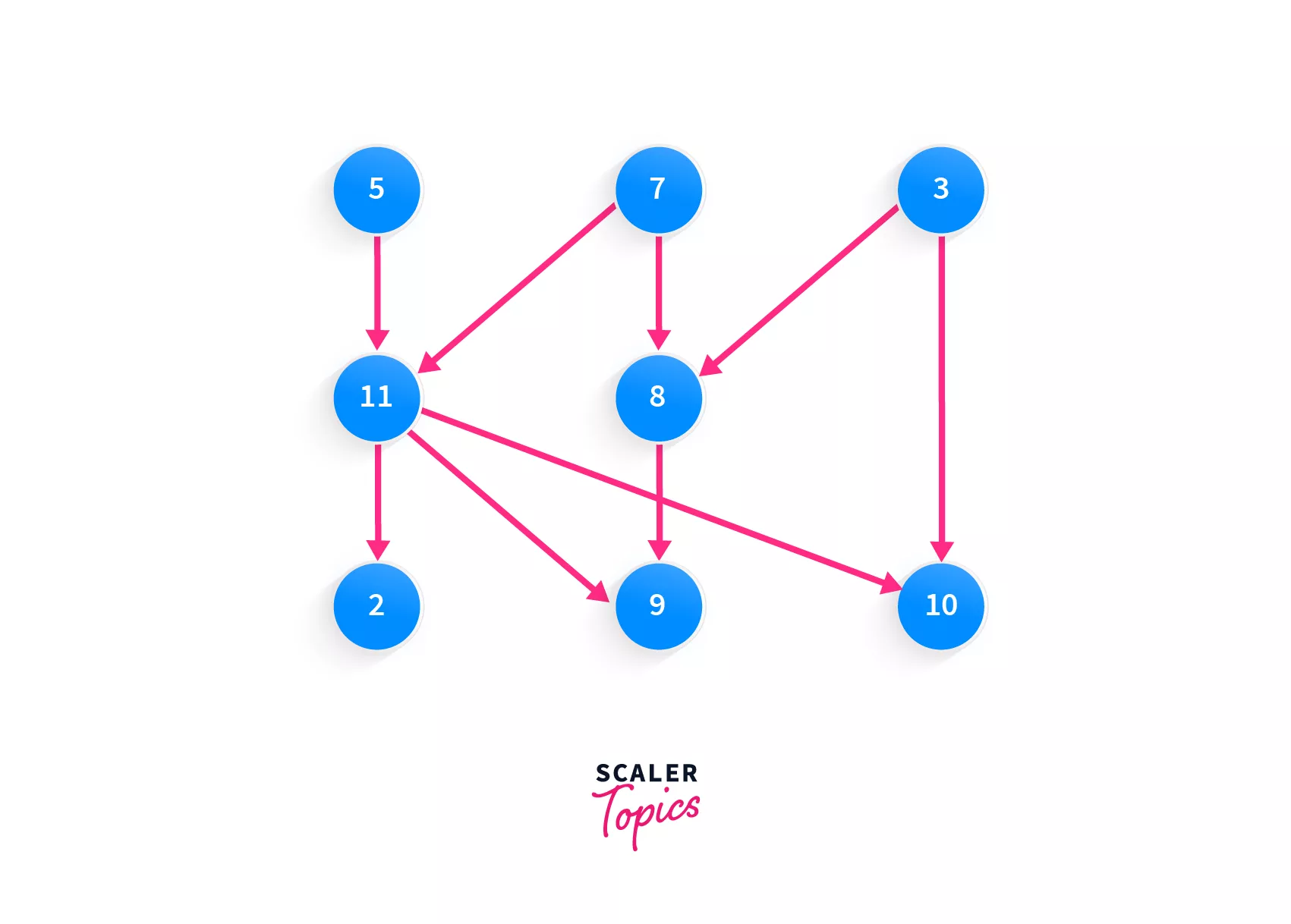Let's take a look at this array - [5, 7, 3, 11, 8, 2, 9, 10]. Do you think this array represents the topologically sorted graph? Yes of course! You can picture this array as moving across the graph from top-to-bottom and left-to-right.

What about this one - [3, 5, 7, 8, 11, 2, 9, 10]? Yes, this one is valid too. As in our previous example, we had two nodes 2, 3 which had the same priority, and the same way here, the nodes 5, 7, 3 and 8, 11 have the same priorities!

How about [7, 5, 11, 3, 10, 8, 9, 2]? Check it for yourself, it's valid.

The last one - [10, 5, 7, 3, 8, 11, 2, 9]? This one isn't valid. Why? Even though the nodes with the same priorities are together, there's one that isn't. The node with the value 10. 10 can not be added to our sorted array before any of the elements that point to it, i.e. 3. 3 must be added to the array first, and only then will 10 be a valid consideration.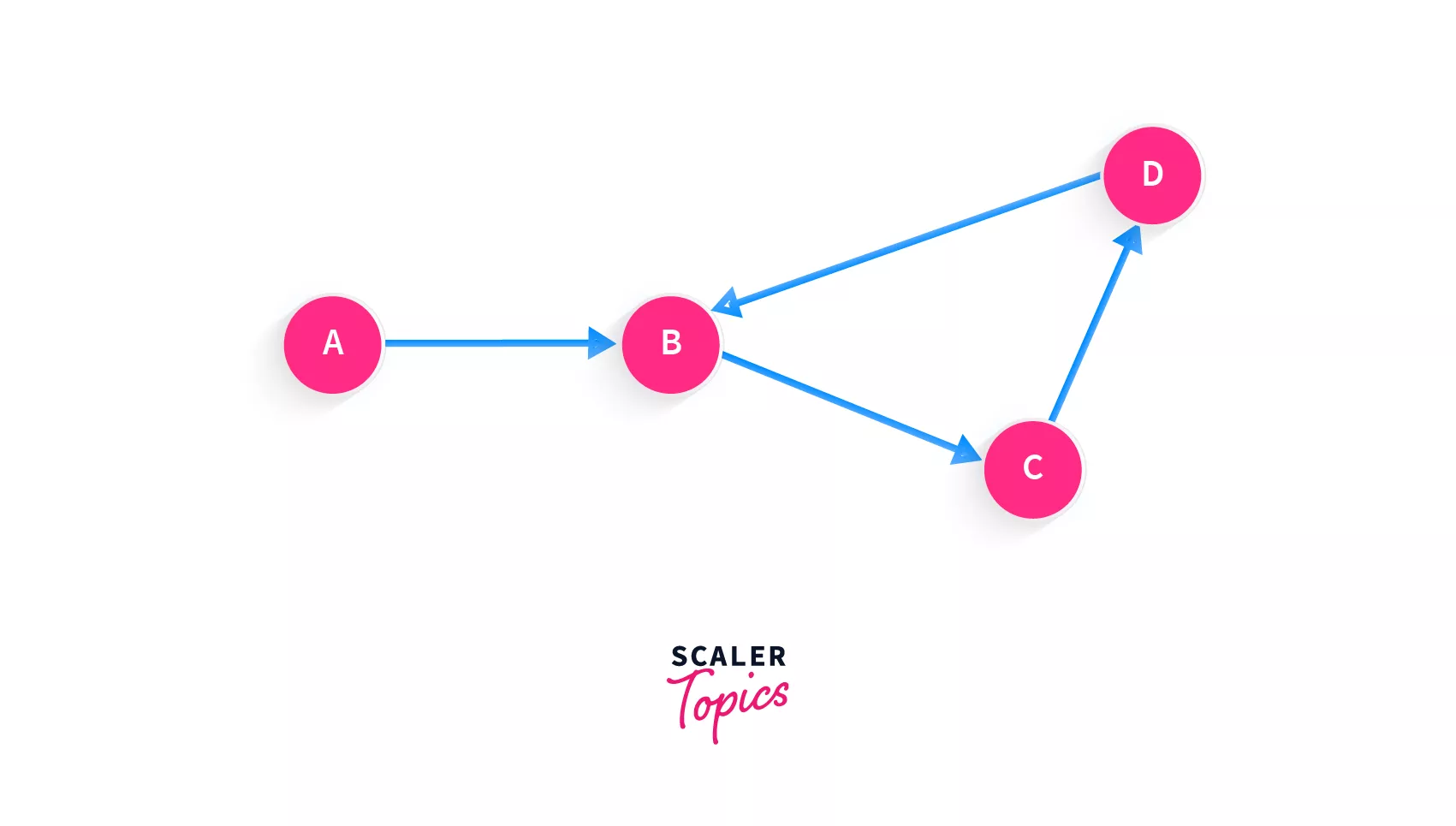As you can see, that this graph has a cycle. Say we add node A to our list initially, and since A points to B, the next node that can be added is B. But not so fast, we see that there's another node that points to B, that is D. So maybe we can add D. Yet again, there's another node that points to D, that is C.

This cycle prevents us from being able to find any correct topological sorting for this graph.

We can now conclude 2 points:

• There can be multiple topologically sorted arrays of a graph
• Directed Cyclic graphs do not have a topologically sorted order.

## Algorithms to Find Topological Sorting

To find the topologically sorted order of any graph, we have 2 algorithms that can be used - Kahn's Algorithm, Depth-first search. In this article we'll discuss the Kahn's algorithm in detail.

## Kahn's algorithm

The first and one of the most popular algorithms that can be used to return the topological sorting of a graph is the Kahn's algorithm. How does it work?

You know how adding a node to our final array that we return, depends on whether or not the nodes pointing to it have been added? Basically it's indegree?

Indegree of a node is the count of incoming edges for that particular node.

Kahn's algorithm basically looks for the nodes that do not have any incoming edges, or have indegree = 0, and then removes it's outgoing edges, making it's outdegree also equal to 0.

Here's the algorithm step by step:

1. Find a vertex that has indegree = 0 (no incoming edges)
2. Remove all the edges from that vertex that go outward (make it's outdegree = 0, remove outgoing edges)
3. Add that vertex to the array representing topological sorting of the graph
4. Repeat till there are no more vertices left.

Let's apply these steps to a graph: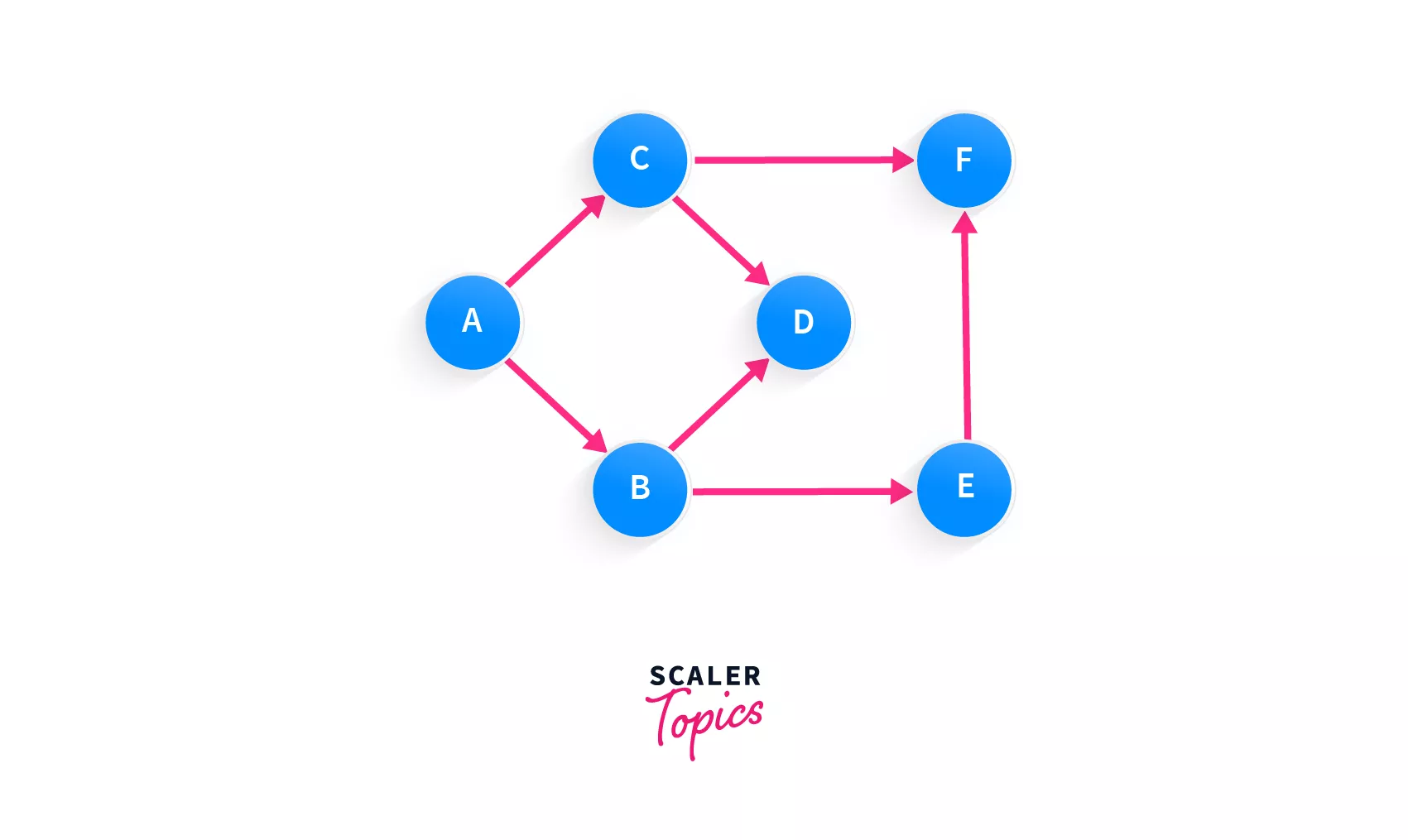What do you think is the first node we must process? Yes, it's A. A is the only node in the graph that has no incoming edges, and has two outgoing edges. So, we're going to isolate this node, by removing it's outgoing edges and adding it to our topological sort array.

Our sorted order of the graph looks like this: [A]

And our graph looks like this now: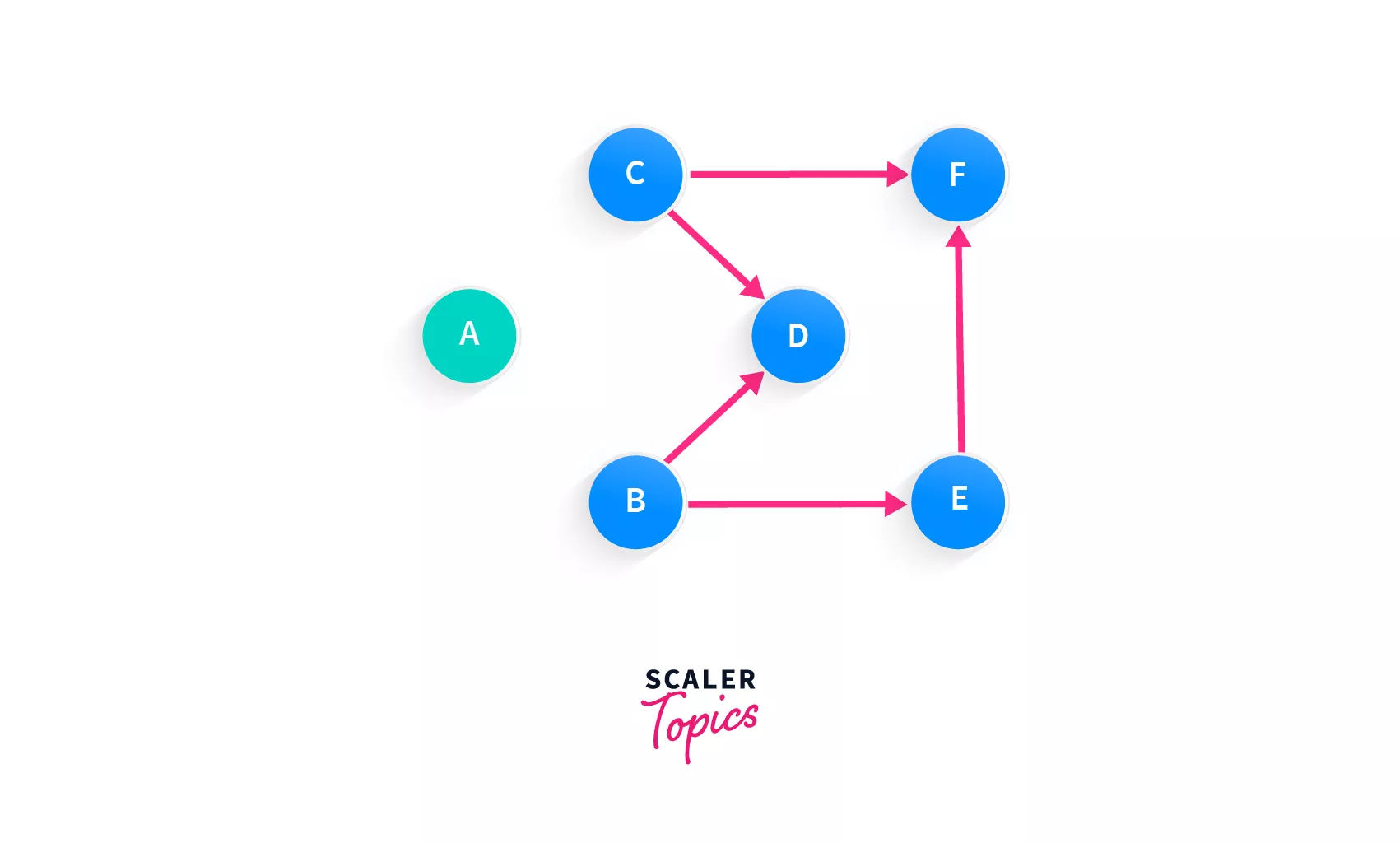The next node that we can add is either B or C. Look closely, none of them have any incoming edges. We know that there can be more than one topologically sorted order of a graph, so we'll just move forward with adding any of the nodes B or C to the array since both of them are equally capable of being added.

Array: [A, C]

Graph: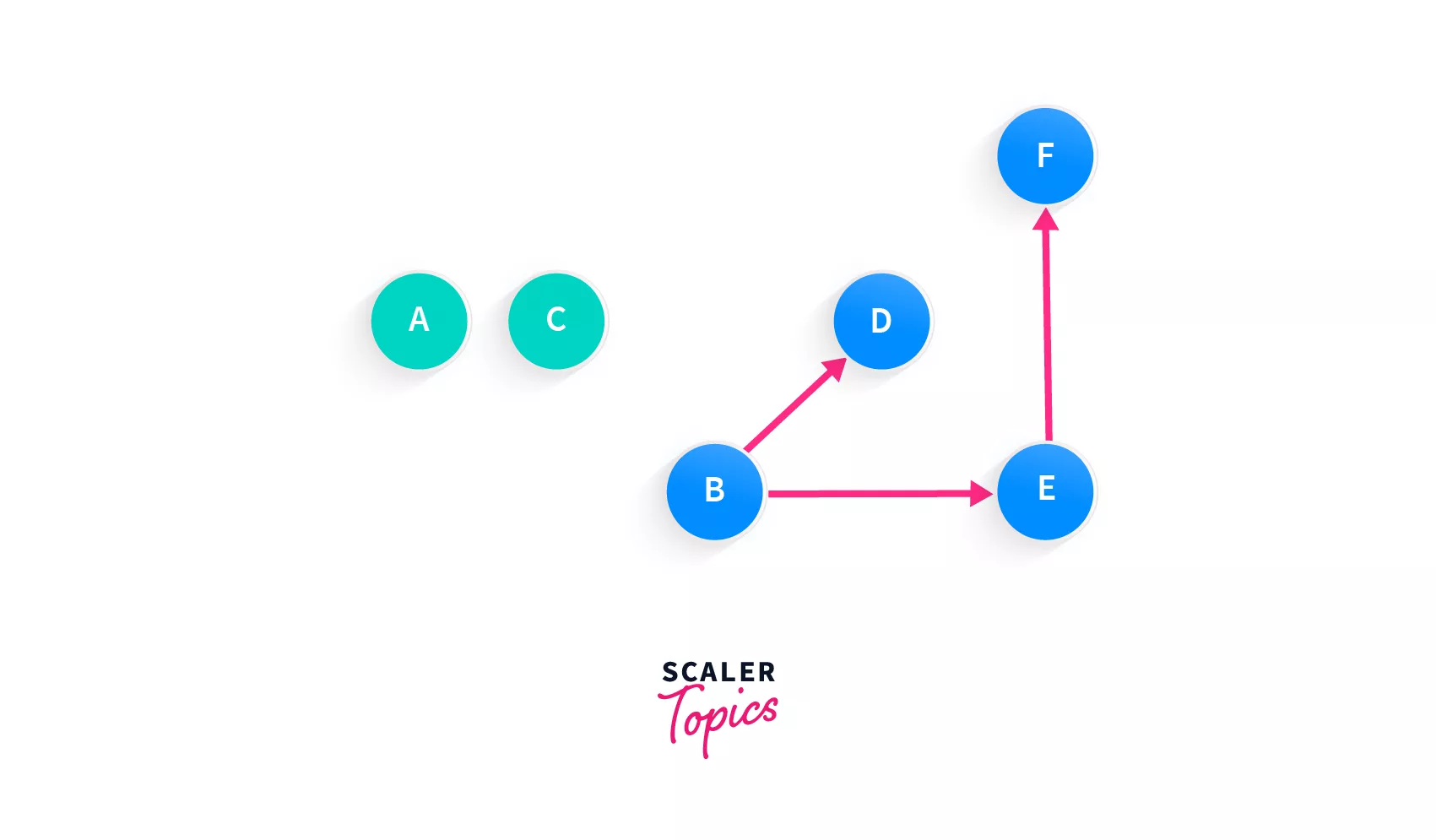Now every node except for B has an incoming edge, and so the next node in our array must be B.

Array: [A, C, B]

Graph: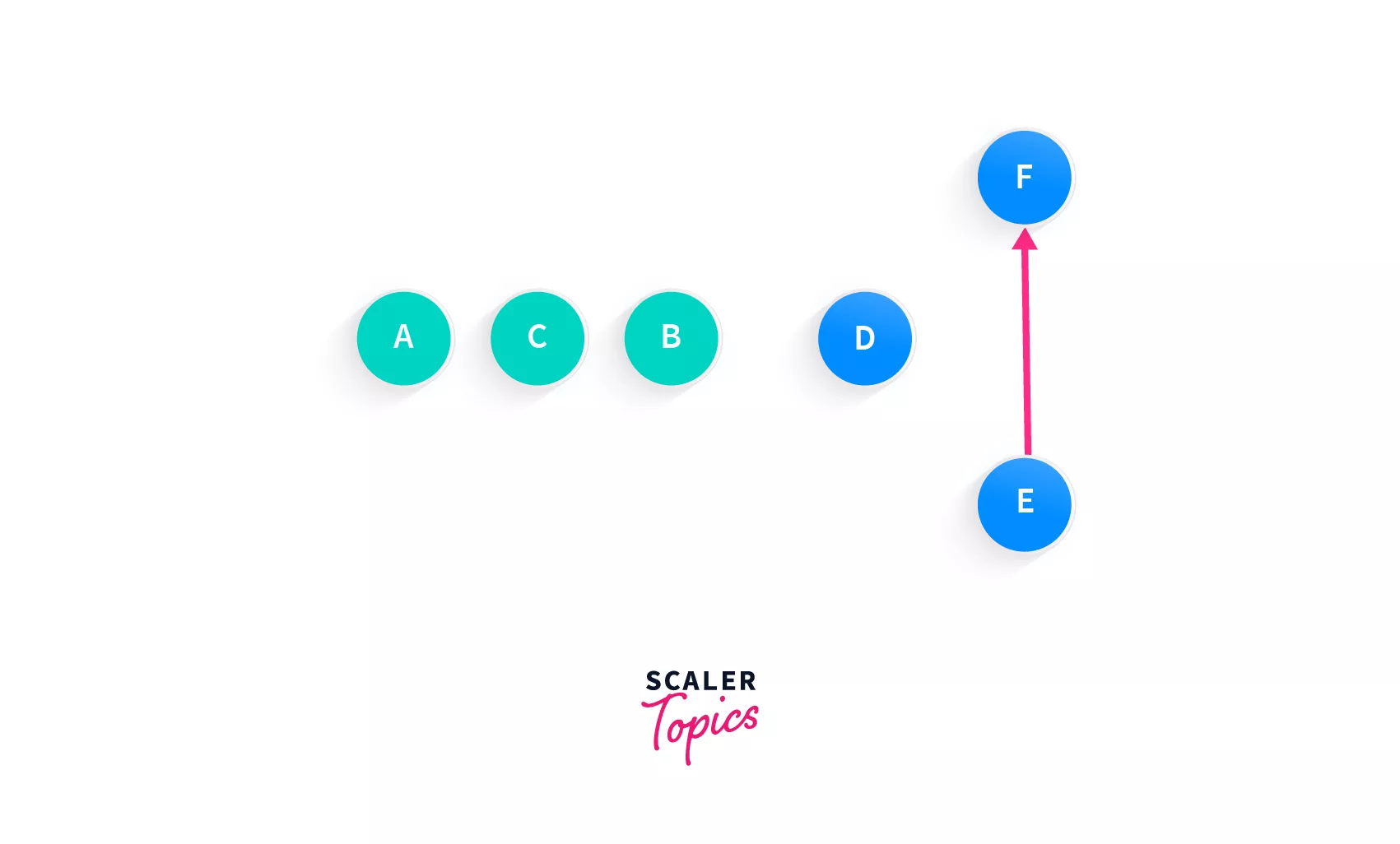The next eligible node is easily D. Although, we can even add E, but since the node is completely isolated, let's go with that one. Remember, a graph can be ordered topologically in multiple ways. Whether you pick D or E right now, will not affect the ordering.

Array: [A, C, B, D]

Graph: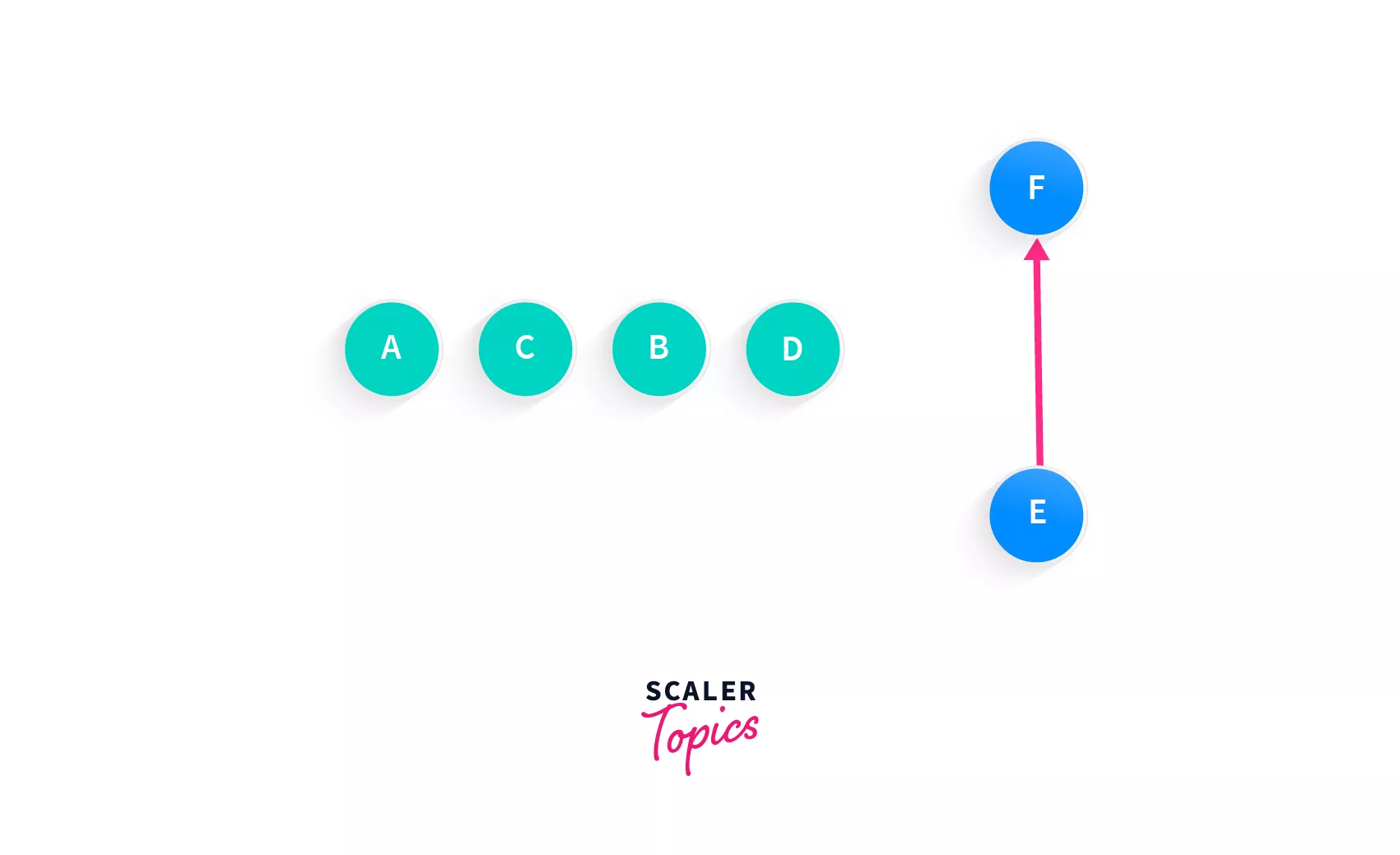E is the only node with no incoming edges, so let's add that, and then the only remaining node will be F.

So, Array: [A, C, B, D, E, F]

And that's our topologically sorted order for this graph using Kahn's algorithm!

Now let's look at some pseudocode before implementing the real code of the Kahn's algorithm.

sorted <-- Empty list initially, of the topologically sorted elements
zero_incoming <-- set of nodes with indegree = 0

while zero_incoming is not empty do:
remove a node n from zero_incoming
add n to the sorted list
for each node out with an edge from n to out do:
remove edge from graph
if out has no other incoming edges then:
insert out in zero_incoming

if graph has edges then:
return Error  {graph has at least 1 cycle}
else
return sorted {our final array}


All the steps we did above for that graph, have been implemented above in pseudocode. Now for the purposes of implementation of the algorithm to better our understanding we have said that our zero_incoming is a set, however, in code we will implement it with a queue because of it's First In First Out properties.

Now before we code it, there's a small change we would make to the algorithm. Instead of actually removing the edges, we would store the edges in an indegree array an instead make the changes to that array.

We're going to create the indegree array by traversing over the adjacency list that we recieve in the input and increasing the count of the neighbour of a node by 1, every time we encounter a node's neighbours (we'll get to the explanation soon).

So when we want to remove the outgoing edges from a particular source node, we simply remove the incoming node that connects to the destination nodes from the source node.

Another algorithm that is used for finding topological ordering of a graph is DFS.

## Depth-first Search for Topological Sorting

To know about the basic DFS algorithm, please google and read about it. In this article, we're only going to give you an idea of how to use the DFS algorithm.

In the depth first search algorithm, we mark the current node that we are at as either visited, or we print it, or we make some modifications to it according to the problem at hand, and then we move on to recursively call the same DFS function on it's adjacent vertices. So how is this related to topological sort?

Here, in topological sort, we want to append the vertex to our sorted array right before it's adjacent vertices, and push nodes that point to other nodes, before the neighbouring nodes that it points to.

The output of the DFS of a graph can definitely be different from it's topological ordering, since in DFS, two neighbours that do not have the same priority might be pushed into the resultant array, but we can not allow that to happen in topological sorting.

However, the depth first search algorithm can be modified to fit our purpose. Here, we're going to use a temporary stack and instead of adding the current vertex to our output, we're going to first call the topological sorting function recursively on all it's adjacent nodes and then push it to the stack.

Now that you have an idea of how to use DFS, code it up in a programming language of your choice!

## Code Implementation - Kahn's Algorithm for Topological Sort

We have seen the pseudocode for Kahn's algorithm for topological sort, let's now get to some real code.

Reiterating Kahn's algorithm, we're going to find out which node has no incoming edges, add it to our sorted list and delete / store the edges in an indegree array and remove the outgoing edges from that node.

Once we're done with that, we would again start our search for a node with indegree = 0. If we fail to find any node with an indegree that is equal to 0, then we can conclude that the graph has a cycle.

### C++

Let's take the code step by step. Scroll up, take a look at the pseudocode once again. Here we're assuming that the input of the Graph is in the form of an adjacency list, and the list will be given in the input of the function.

The first two lines of the pseudocode were:

sorted <-- Empty list initially, of the topologically sorted elements
zero_incoming <-- set of nodes with indegree = 0


These were the first two steps but here we're just going to tweak them a little bit. The sorted list is going to be vector, and the zero_incoming will be a queue because of it's first in first out properties.

Since we do not wish to modify the graph (modifying the input isn't a good practice unless required), or delete it's edges, we will create another data structure, a vector, to store the indegrees of every node, initially all 0. Indegree of a node is the number of incoming edges in the node.

The variable v in the following code is the number of vertices.

vector<int> sorted;
vector<int> indegrees(v, 0);
queue<int> zero_incoming;


The next step would be to store all the indegrees (number of incoming edges) of the nodes in the array. For this purpose, we will use an iterator. An iterator will allow us to look at every element in the list one at a time.

list<int>::iterator itr;


So itr is the name of our iterator in the above code, for the list<int> template class. In iterators we use list<int>.begin() and list<int>.end() which give us instances that point to the beginning and end of the list respectively.

When we're going through all the neighbours of a node, we will just use the begin() and end() instances of the node's corresponding list of neighbours to traverse it and store it's length as number of incoming nodes.

In short, we're going to traverse the adjacency list using the iterator, and store the indegrees (number of incoming edges of a node) of all nodes in the indegrees vector.

for (int node = 0; node < v; node++){
list<int>::iterator itr;
for (itr = adj_list[node].begin(); itr != adj_list.end(); itr++){
indegrees[*itr]++;
}
}


Now that we have populated the indegrees array, the next step is to insert the nodes with indegree = 0 into our queue.

for (int i = 0; i < v; i++){
if (indegrees[i] == 0)
zero_incoming.push(i);
}


This way, in our queue we have all nodes with indegree = 0 and we can now proceed to process them.

Remember that in topological sorting, we might not always receive an order. There could be a cycle in the graph and that can only be detected if the number of nodes in the sorted array are not equal to the total number of nodes in the complete graph or in other words, the number of nodes visited and processed will be different from the total number of nodes in the graph.

So, to keep a count of the visited nodes, we will initialize another variable that stores them. At the end of the program, if the total number of nodes is the same as the number of nodes that have been visited, we know that there isn't a cycle in the graph.

int visited_nodes = 0;


We now need to process the nodes that are there in the queue - zero_incoming.

while (!zero_incoming.empty()){
int node = zero_incoming.front();
zero_incoming.pop();
sorted.push_back(node);
...
}


In the above code, we started a loop that will run till the queue is empty. We stored the front of the queue in an integer called node, and then popped it from the queue. Since we know that it does not have any incoming edges, we add it to our sorted array.

Can you guess what should come in place of the ...?

Yep! You're right, we now need to process it's neighbours. We will decrease the indegree of all the nodes that it pointed to by 1 (this is same as removing all outgoing edges when you think of it).

list<int> iterator itr;
for (itr = adj_list[node].begin(); itr != adj_list[node].end(); itr++){
if (--indegrees[*itr] == 0)
zero_incoming.push(*itr);
visited_nodes++;
}


That's it! Now we check if the visited nodes is equal to total number of nodes then we can simply return the sorted vector, and if not, we return an error saying there's a cycle in the graph.

Here's the complete code:

vector<int> topological_sort(vector<list<int>> adj_list){
int v = adj_list.size();
vector<int> sorted;
vector<int> indegrees(v, 0);
queue<int> zero_incoming;

for (int node = 0; node < v; node++){
list<int>::iterator itr;
for (itr = adj_list.begin(); itr != adj_list.end(); itr++){
indegrees[*itr]++;
}
}
int visited_nodes = 0;
while (!zero_incoming.empty()){
int node = zero_incoming.front();
zero_incoming.pop();
sorted.push_back(node);
list<int>::iterator itr;
for (itr = adj_list[node].begin(); itr != adj_list[node].end(); itr++){
if (--indegrees[*itr] == 0)
zero_incoming.push(*itr);
visited_nodes++;
}
}
if (visited_nodes != v)
throw "Graph contains a cycle";
else
return sorted;
}


At the end of the code, we have simply added an error if the number of visited nodes is not equal to the total number of nodes in the graph.

For your reference, here's the code in Java:

### Java

public static int[] topological_sort(int[][] adj_list){
int v = adj_list.length;
int sorted[] = new int[];
int indegrees = new int[v];
Queue<Integer> zero_incoming = new LinkedList<Integer>();
for (int i = 0; i < v; i++){
ArrayList<Integer> temp = (ArrayList<Integer>)adj_list[i];
for (int node : temp)
indegrees[node]++;
}
for (int node = 0; node < v; node++){
if (indegrees[node] == 0)
zero_incoming.push(node);
}
int visited_nodes = 0;
while (!zero_incoming.empty()){
int node = zero_incoming.poll();
for (int u: adj_list[node]){
if (--indegrees[u] == 0)
}
visited_nodes++;
}
if (visited_nodes != v)
throw new Exception("Graph contains a cycle!");
else
return sorted;
}


You can now write the code in any other language you want!

## Time Complexity Analysis

To analyze the time complexity of this algorithm (Kahn's algorithm), we're going to break the Kahn's algorithm down into small parts.

1. Determining the indegree of each node - O(M). Here M is the number of edges. Why? To determine the indegree, we would have to look at every directed edge in the graph once.
2. Finding nodes with 0 indegree - O(N). This is a simple operation in which we just check which nodes have no incoming edges by looping through all the nodes. Here, N is the number of nodes.
3. Add all nodes to sorted array. This loop could be running once for every node which is O(N) times. In the body of this loop we perform one of two operations
• add the node to our sorted array
• decrease the indegree of it's neighbours by 1. We will decrement only once for every edge (obviously) and hence this step becomes O(M) where again M is number of edges.
4. Checking if we have included all the nodes of the graph into the array or if we have found a cycle : O(1)

This way, we can see that the total time complexity of the algorithm is O(N + M).

Let's also discuss the space complexity of the Kahn's algorithm. We created the following data structures in our code:

• Nodes with zero indegrees - O(N)
• Sorted array - O(N)

Hence the final space complexity of the algorithm is - O(N)

## Applications of Topological Sort Algorithm

While reading the article above, you definitely must have thought of some of the places where you could use this algorithm. Let's go through some of it's very common uses:

### 1. Finding Cycle in a Graph

We saw above, that when there is a cycle in a graph, there is no topological sort / topological order of a graph possible. Suppose we have certain processes, process 1, 2 and 3. We need to find the right order to execute them in. The information that you are given is:

• To complete process 1, you must complete process 2
• To complete process 3, you must complete 1
• To complete process 2, you must complete 3

Here's how the graph created would look like: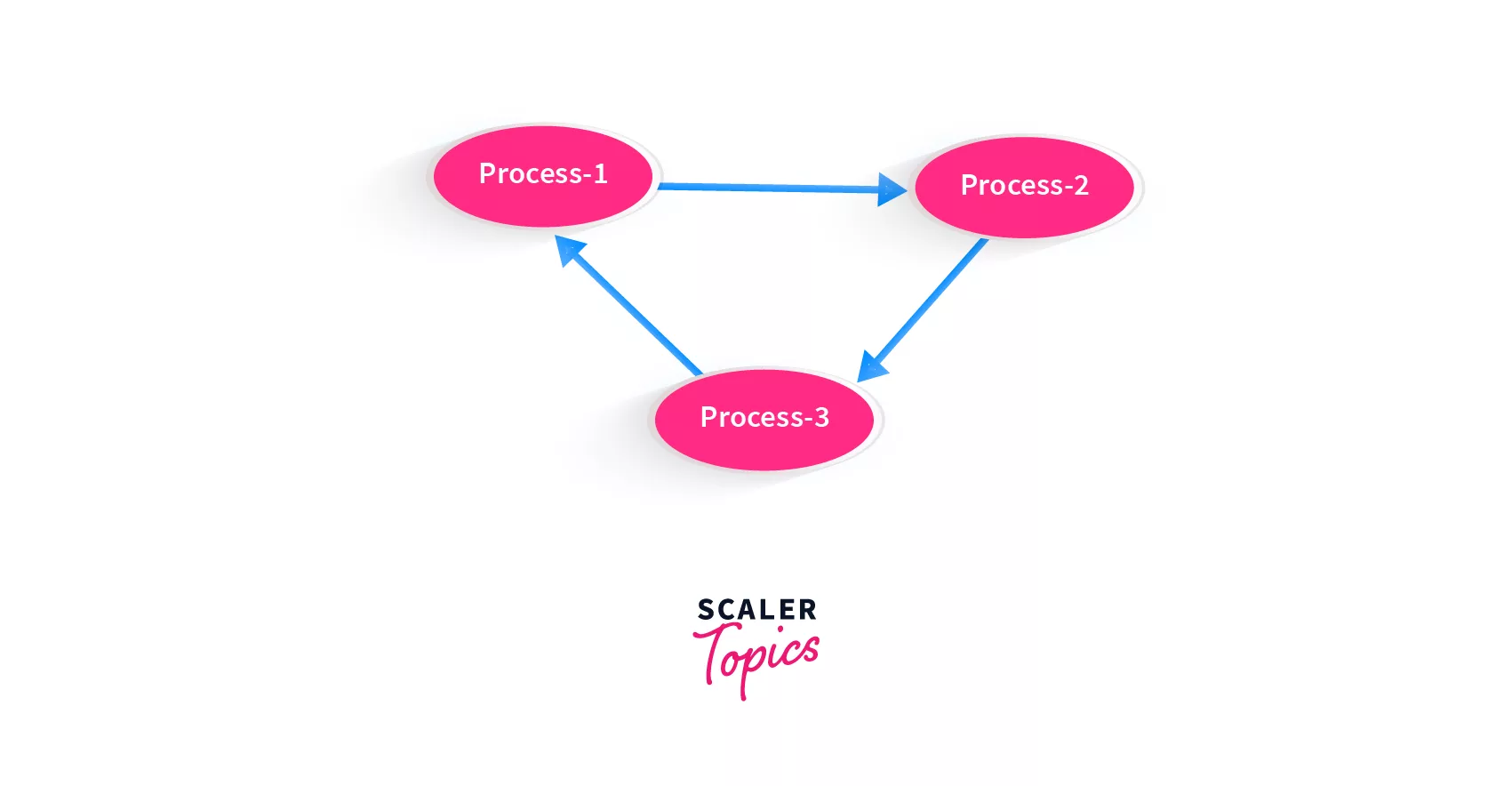This graph contains a cycle!

How would the computer know whether it's a cycle or not? It will get stuck in the cycle trying to find the process that should be executed first!

Using topological sort, we can detect if the graph contains a cycle, because at least one of the nodes will break the topological order. If we are able to find out that our processes input contains a cycle, we will not feed it to the system!

### 2. Operating System Deadlock Detection

In the operating system, we have multiple processes and states that are waiting to be executed, or have already been executed.

Say there is a process that is currently waiting to be executed because it needs some resources from another process. But if the process that will provide resources, is itself in a waiting state, holding the resource, then how will any process be executed? This state is called a deadlock.

If you noticed, this too sounds like a cycle in the graph, and as we saw above topological sort is the best way to detect a cycle and prevent a deadlock!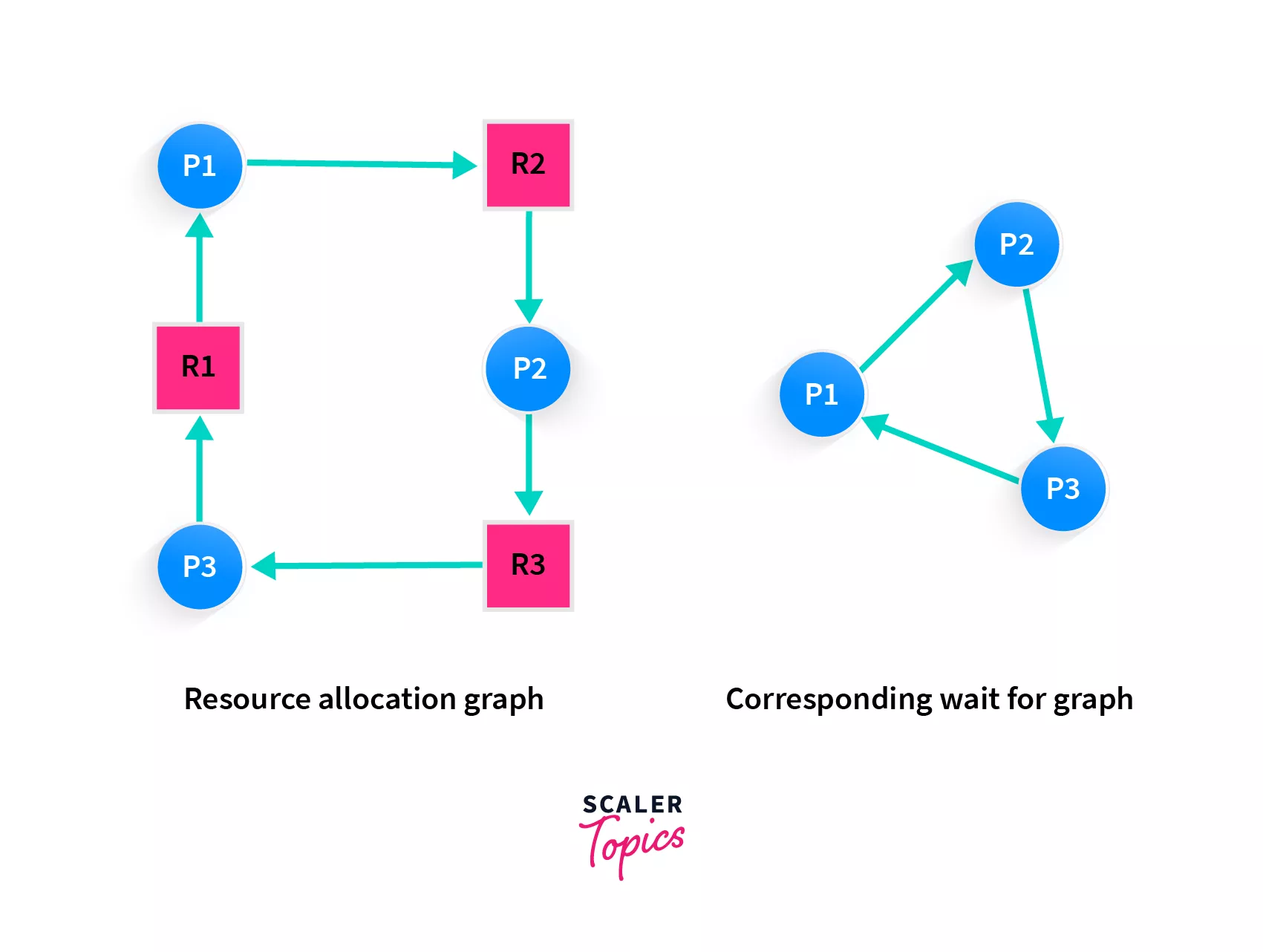Here P1, P2 and P3 are processes, and R1, R2 and R3 are resources. In a deadlock situation, it becomes just like a cyclic graph and topological sort is used in operating systems to prevent a deadlock, i.e. by first detecting it.

## Practice Problem Based on Topological Sort

A very common problem that uses topological sort is the Course Schedule problem.

The problem statement goes like this: You are given a list of courses that you have to take in your university. Each course has some or zero prerequisites. Your task is to determine whether you can complete the courses or not.

For a moment, let's forget about the input or the output of the problem, focusing only on the problem statement.

Let's take an example, you have courses 1, 2, 3, 4, 5, 6. To complete course 3, you must have already completed course 2 and 5. For course 4, you should have done course 1. For course 6, you should have completed 3, 4 and 5.

Hold on, do you see any similarity between these above statements and our cake discussion? While baking a cake as well, we needed to complete certain steps before moving on to the next, and that is the same issue here! We have to complete some courses to go on to study the next or the rest of the ones remaining. Can we solve this using topological sorting? How can topological sorting be helpful?

Look at the problem statement again, you need to find out whether you can complete all the courses or not. In this current example, you can complete all the courses in order.

So when or what is the condition when you can not complete the courses? Take a moment to think. Say you had another intermediate course addded, course 7. Prequisite of course 7 is course 3, and also, before you complete course 5, you must complete course 7.

Do you think you can take the courses? Let's make it easier by representing it on a graph. You should be comfortable with that by now!

Initially, when we only had courses 1 through 6, here's what it looked like: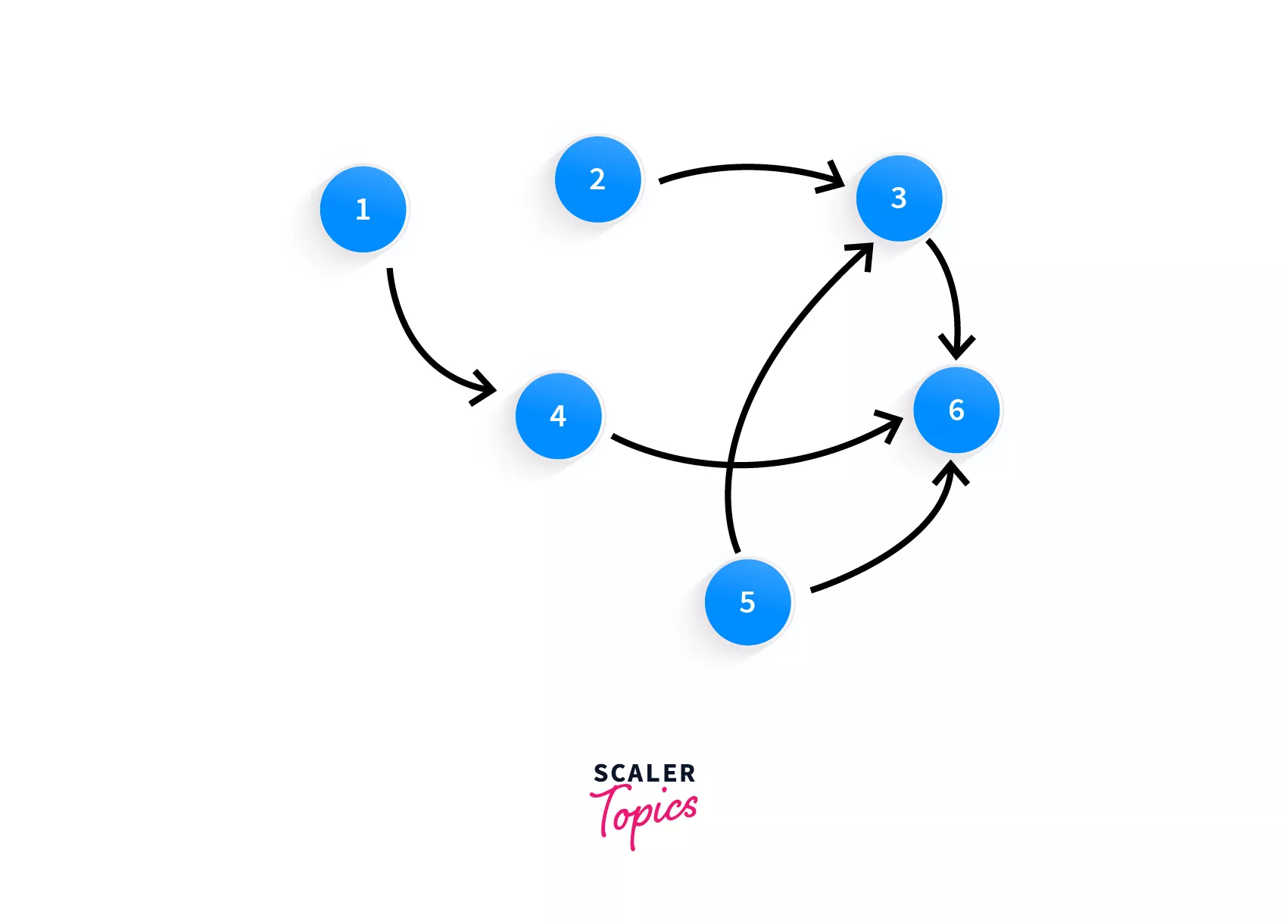With the addition of course 7 and the prerequisites of 7 and 5, the graph now looks like this:Looking at the graph, surely the first thing that you must have looked for is a cycle, because that's what we've been doing all along in this article. Did you spot it?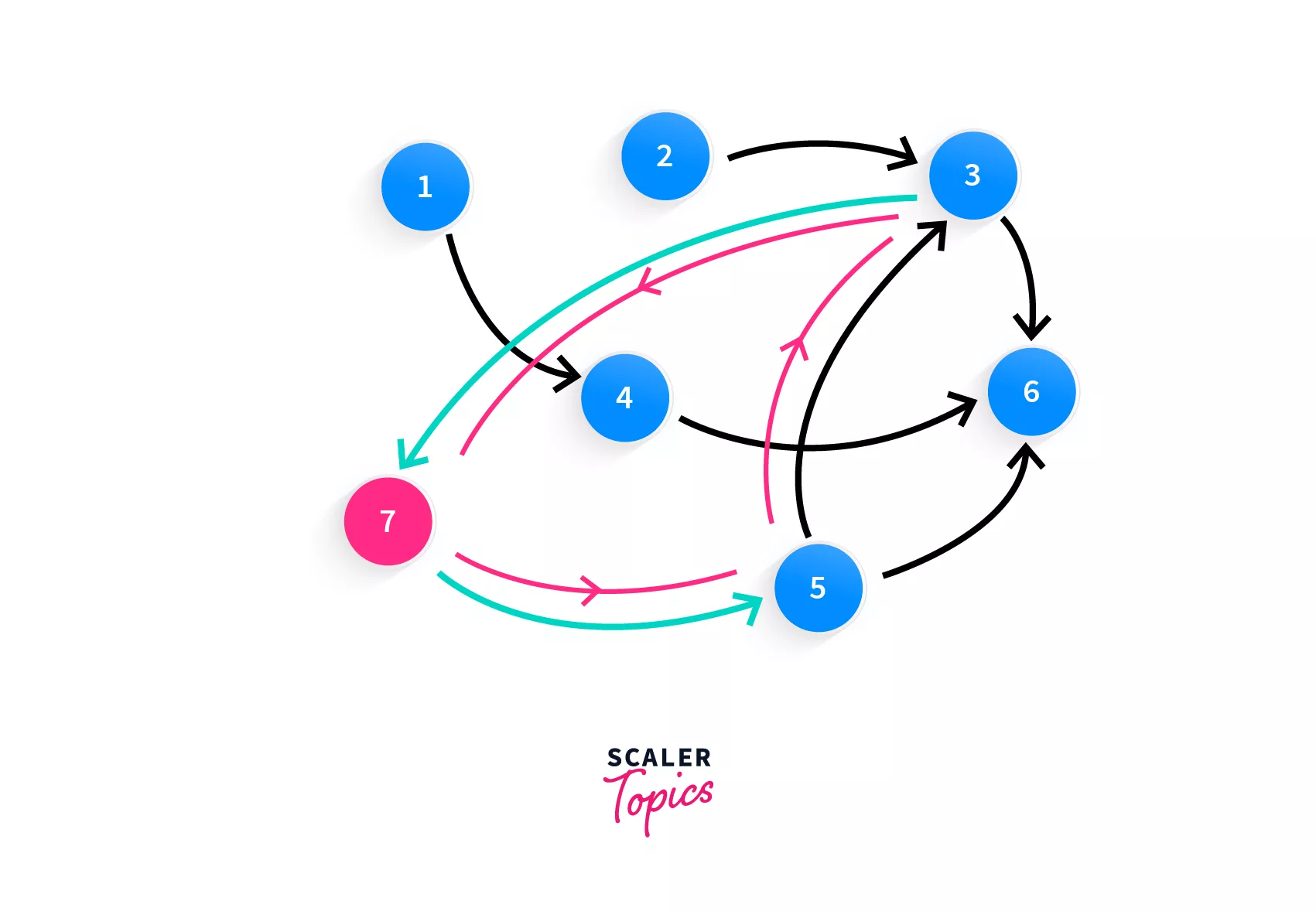Follow the blue line, that's your cycle! Course 7 requires the completion of course 3, while course 3 requires completion of course 5 and course 5 requires course 7!Now you have spotted the problem, you cannot take these courses. Have you now been able to deduce the problem? We simply have to check if there's a cycle in the graph.

Let's also see the input and output form, you will be given a list of pairs such has [a, b] where a,b means that to complete course a, course b is a prerequisite. Input given for the above graph (without the cycle) would be:

[[4, 1], [3, 2], [3, 5], [6, 4], [6, 5], [6, 3]]

And the output would be True, since there is no cycle in this graph and you can take all the courses.

You are already a pro at topological sort, so the only piece of code that needs to be added to the function we created earlier (Kahn's algorithm), is another function that creates the adjacency list for us and once we feed it to the topological_sort function, we're done!

In C++:

// declare a list of lists called graph
vector<vector<int>> graph;
// traverse the input - prerequisites
for (auto& e: prerequisites){
graph[e].push_back(e);
}


With this short 3 line code, you have now created your adjacency list which you can give to the topological sorting function and that's that!

## Conclusion:

1. Topological Sort is an algorithm which sorts a directed graph by returning an array that consists of nodes where each node appears before all the nodes it points to.
2. There can be multiple topological orderings of a graph
3. Two algorithms to find the topological ordering of a graph:
• Kahn's algorithm - find nodes with zero indegree, add them to sorted array, delete outgoing edges and continue.
• Modified DFS - visit node, mark as visited, process neighbours (nodes it points to), if none then add to sorted array. If it had neighbours then add them to stack, and continue popping and processing.
4. Time complexity of Kahn's algorithm - O(N + M) where n is number of nodes and m is number of edges.
5. Space complexity of Kahn's algorithm - O(N)
6. Applications:
• Finding Cycle in Graph
• Operating system deadlock detection
• ... and more
Challenge Time!Time to test your skills and win rewards! Note: Rewards will be credited after the next product update.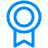Certificates
Data Structures Tutorial
This program includes modules that cover the basics to advance constructs of Data Structures Tutorial. The highly interactive and curated modules are designed to help you become a master of this language.'
If you’re a learning enthusiast, this is for you.Criteria
Upon successful completion of all the modules in the hub, you will be eligible for a certificate.
You need to sign in, in the beginning, to track your progress and get your certificate.
Free Courses by top Scaler instructors